IT技術を分かりやすく簡潔にまとめることによる学習の効率化、また日常の気付きを記録に残すことを目指します。

# Valgrindで行番号を表示させる方法

## (１) Valgrindで行番号を表示させる方法

（Before）
==XXXXX==    by 0x40059E: main (in /tmp_rainbow/memorytest)


（After）
==XXXXX==    by 0x40059E: main (memorytest.cpp:5)


### (１-１) 行番号を表示させる方法

（実行コマンド）メモリチェック
valgrind --tool=memcheck --leak-check=yes ../memorytest


これは、C++のプログラムをgccでコンパイルする際に「-g」オプションを指定していないと、チェック時に問題となっている正確な行番号が表示されません。

（例：BEFORE）※発生したmainの行番号が分からない
==21696== HEAP SUMMARY:
==21696==     in use at exit: 100 bytes in 1 blocks
==21696==   total heap usage: 1 allocs, 0 frees, 100 bytes allocated
==21696==
==21696== 100 bytes in 1 blocks are definitely lost in loss record 1 of 1
==21696==    at 0x4C2C866: operator new[](unsigned long) (vg_replace_malloc.c:579)
==21696==    by 0x40059E: main (in /tmp_rainbow/memorytest)
==21696==
==21696== LEAK SUMMARY:
==21696==    definitely lost: 100 bytes in 1 blocks
==21696==    indirectly lost: 0 bytes in 0 blocks
==21696==      possibly lost: 0 bytes in 0 blocks
==21696==    still reachable: 0 bytes in 0 blocks
==21696==         suppressed: 0 bytes in 0 blocks

（図１１１）行番号が表示されない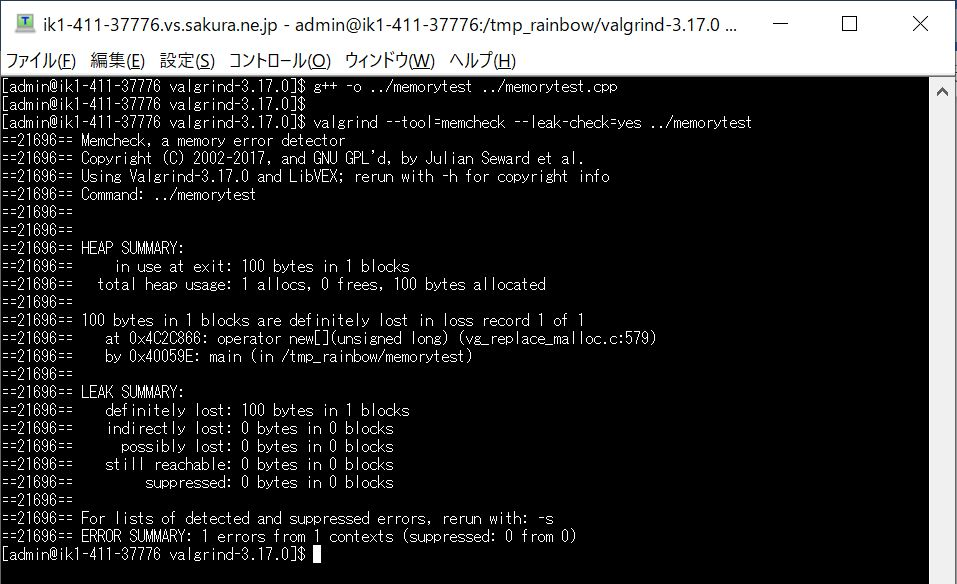しかし、対象のC++プログラムをコンパイルする際に「-g」オプション（デバッグ用情報の生成）を付与すると、行番号が表示されるようになります。

（コマンド）コンパイル時に「-g」付与
\$ g++ -g -o memorytest memorytest.cpp

この状態で次のコマンドでメモリチェックを実行すると、「by 0x40059E: main (memorytest.cpp:5)」のように問題となった具体的な行数を教えてくれます。

（実行コマンド）メモリチェック
valgrind --tool=memcheck --leak-check=yes ../memorytest

（例：AFTER）※行番号の情報「memorytest.cpp:5」がある
==11651== HEAP SUMMARY:
==11651==     in use at exit: 100 bytes in 1 blocks
==11651==   total heap usage: 1 allocs, 0 frees, 100 bytes allocated
==11651==
==11651== 100 bytes in 1 blocks are definitely lost in loss record 1 of 1
==11651==    at 0x4C2C866: operator new[](unsigned long) (vg_replace_malloc.c:579)
==11651==    by 0x40059E: main (memorytest.cpp:5)
==11651==
==11651== LEAK SUMMARY:
==11651==    definitely lost: 100 bytes in 1 blocks
==11651==    indirectly lost: 0 bytes in 0 blocks
==11651==      possibly lost: 0 bytes in 0 blocks
==11651==    still reachable: 0 bytes in 0 blocks
==11651==         suppressed: 0 bytes in 0 blocks

（図１１２）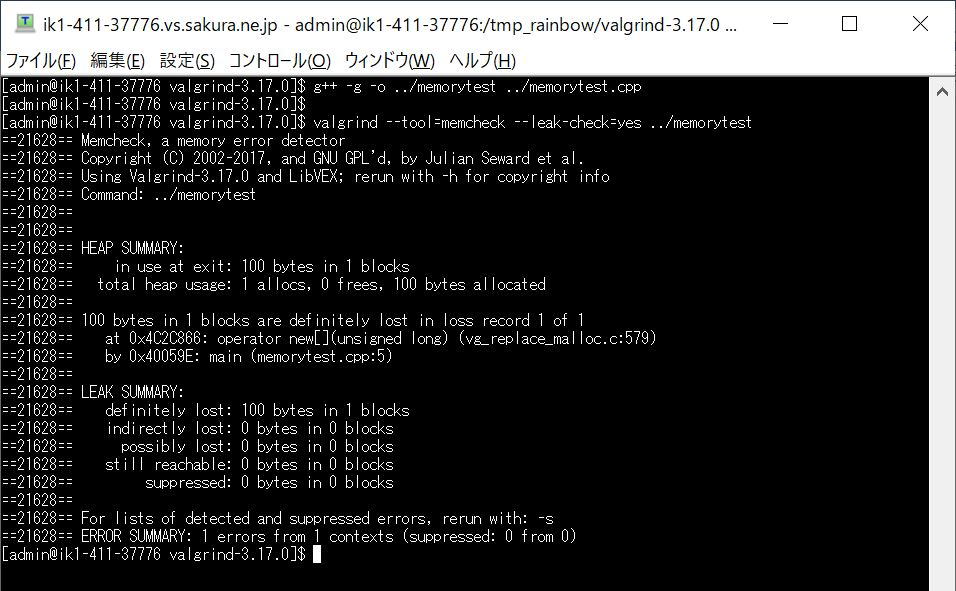### (１-２) （参考）「-g」オプションについて

「-g」を指定してコンパイルすると、実行ファイル自身（拡張子「.out」）の中にGDBデバッガで使えるデバッグ情報を生成・保持します（正確には「シンボルテーブル」（※注１）やデバッグ用のメタデータが作られます）。

それらのデバッグ情報は、実行プログラムの領域とは別の領域に書き込みされるので、デバッガ以外（gdb以外）でプログラム実行（例：本番環境での実行など）する場合においては、性能面等での影響はありません。

（※注１）
「シンボルテーブル」はコンパイラが使用するデータ構造の事で、ソースコード内の各識別子がその定義に関連する情報とともに格納されています（例：スコープ、型、行番号など）。ポインタと参照の違いについてサンプルPGを使ってご紹介

＜目次＞ (１) ポインタと参照の違いについてサンプルPGを使ってご紹介 　(１-１) ポインタと参照の概要 　(１-２) 両者の違い①：宣言／初期化 　(１-３) 両者の違い②：再代入 …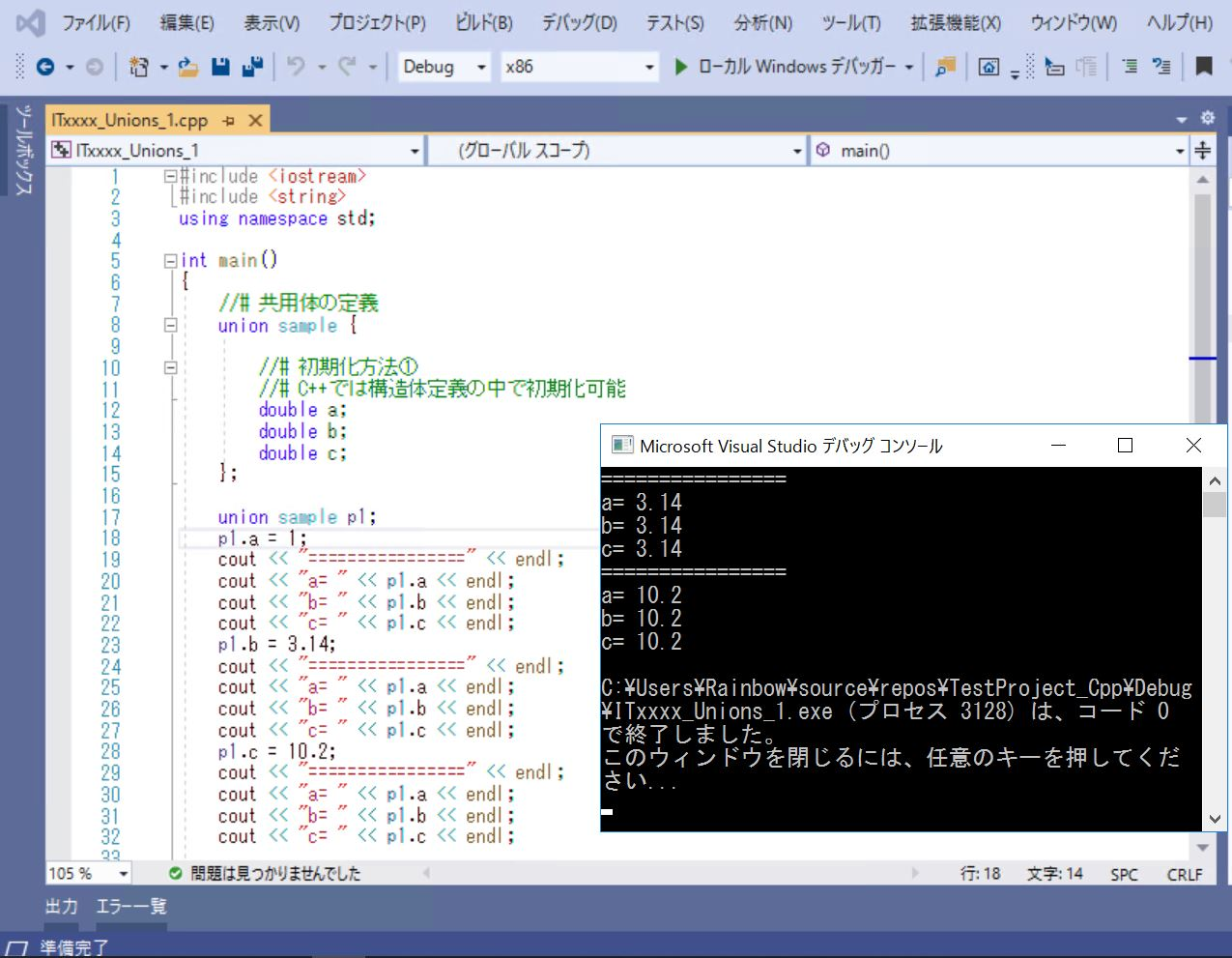C++の共用体とは？構造体との違いや使用方法について

＜目次＞ (１) C++の共用体とは？構造体との違いや使用方法について 　(１-１) 共用体とは？構造体との違いは？ 　(１-２) 共用体の定義方法、変数定義方法、初期化方法 　(１-３ …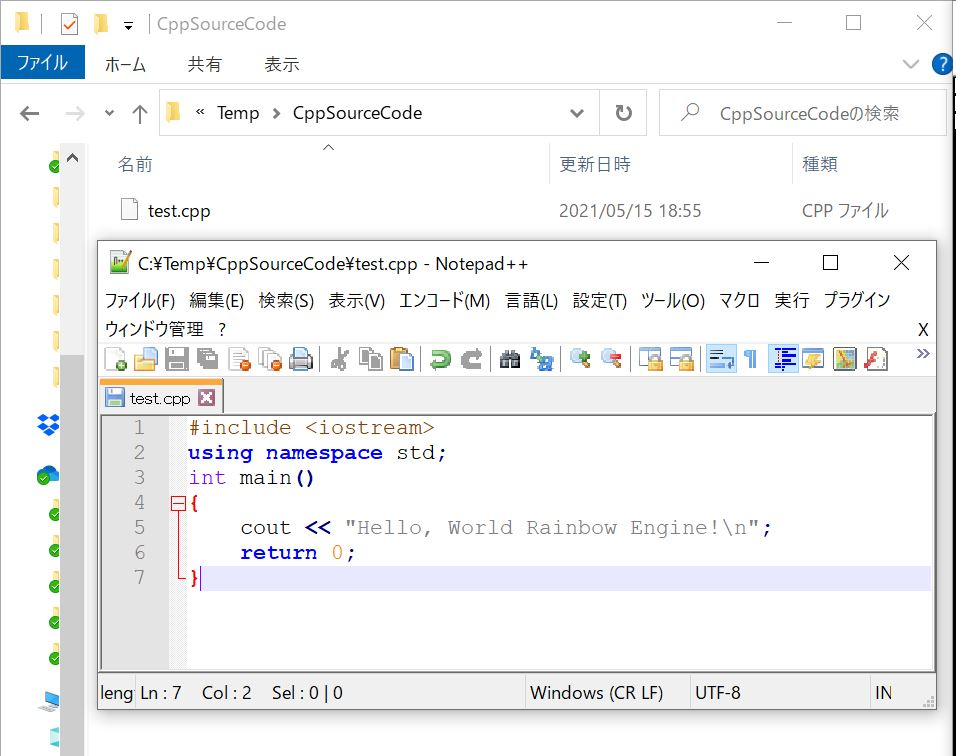C++をLinuxでコンパイルする方法について

＜目次＞ (１) C++をLinuxでコンパイルする方法について 　(１-１) STEP0：【事前準備】ターミナル機能の準備（ターミナル、Teraterm、Putty） 　(１-２) S …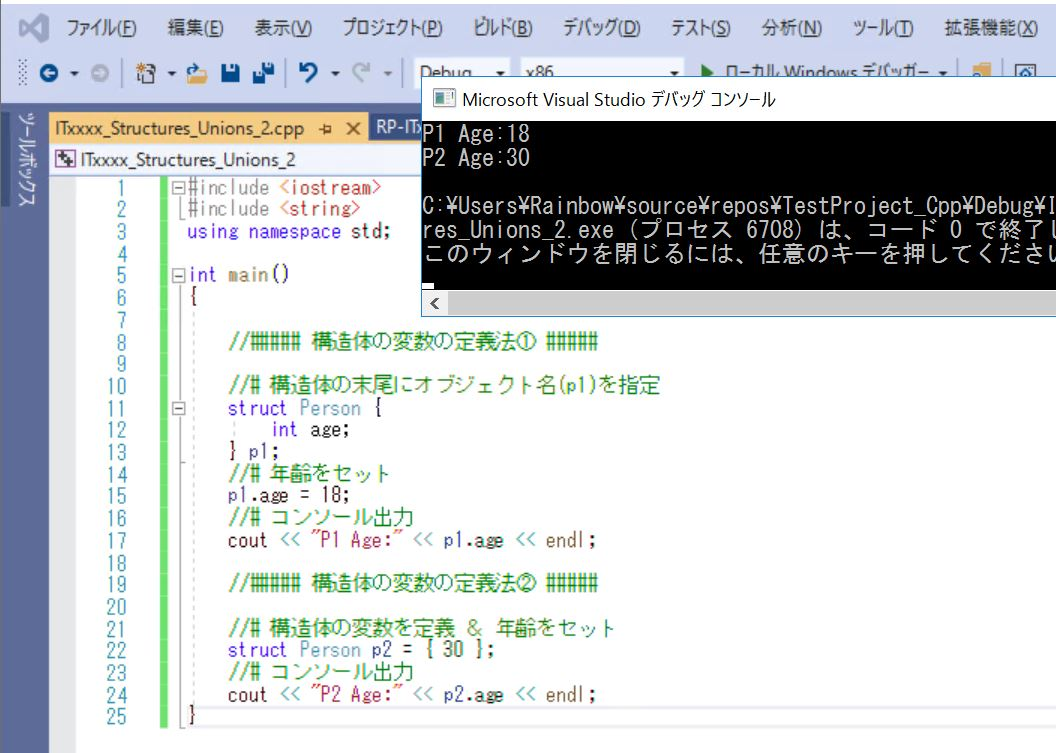C++の構造体とは？の作り方や使い方について

＜目次＞ (１) C++の構造体とは？の作り方や使い方について 　(１-１) 構造体とは？ 　(１-２) サンプルプログラム 　(１-３) 構造体の変数を定義する方法 　(１-４) 構造 …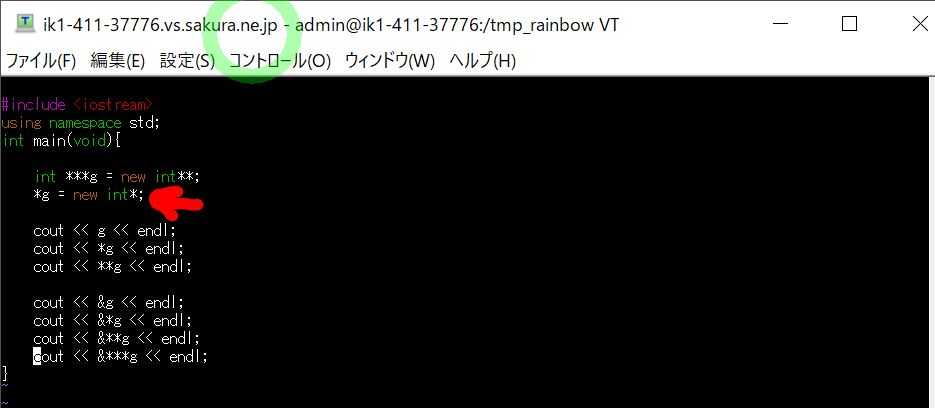C++の「int **g = new int*;」の意味について

＜目次＞ (１) C++の「int **g = new int*;」の意味について 　(１-１) 「int **g = new int*;」の意味 　(１-２) サンプルで理解を深める …

Top